Courses

# Test: Area Of Circles

## 14 Questions MCQ Test Quantitative Aptitude for GMAT | Test: Area Of Circles

Description
This mock test of Test: Area Of Circles for GMAT helps you for every GMAT entrance exam. This contains 14 Multiple Choice Questions for GMAT Test: Area Of Circles (mcq) to study with solutions a complete question bank. The solved questions answers in this Test: Area Of Circles quiz give you a good mix of easy questions and tough questions. GMAT students definitely take this Test: Area Of Circles exercise for a better result in the exam. You can find other Test: Area Of Circles extra questions, long questions & short questions for GMAT on EduRev as well by searching above.
QUESTION: 1

### The length of a rope, to which a cow is tied, is increased from 19m to 30m. How much additional ground will it be able to graze? Assume that the cow is able to move on all sides with equal ease. Use π = 22/7 in your calculations.

Solution:

The cow can graze the area covered by the circle of radius 19m initially, as the length of the rope is 19m.

Area of a circle = π * (radius)2
Therefore, the initial area that the cow can graze =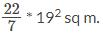When the length of the rope is increased to 30m, grazing area becomes =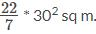The additional area it could graze when length is increased from 19m to 30m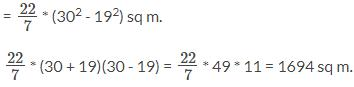QUESTION: 2

### A lady grows cabbages in her garden that is in the shape of a square. Each cabbage takes 1 square feet of area in her garden. This year, she has increased her output by 211 cabbages as compared to last year. The shape of the area used for growing the cabbages has remained a square in both these years. How many cabbages did she produce this year?

Solution:

The shape of the area used for growing cabbages has remained a square in both the years.
Let the side of the square area used for growing cabbages this year be X ft.
Therefore, the area of the ground used for cultivation this year = X2 sq.ft.

Let the side of the square area used for growing cabbages last year be Y ft.
Therefore, the area of the ground used for cultivation last year = Y2 sq.ft.

As the number of cabbages grown has increased by 211, the area would have increased by 211 sq ft because each cabbage takes 1 sq ft space.

Hence, X2 - Y2 = 211
(X + Y)(X - Y) = 211.
211 is a prime number and hence it will have only two factors. i.e., 211 and 1.
Therefore, 211 can be expressed as product of 2 numbers in only way = 211 * 1
i.e., (X + Y)(X - Y) = 211 * 1
So, (X + Y) should be 211 and (X - Y) should be 1.
Solving the two equations we get X = 106 and Y = 105.

Therefore, number of cabbages produced this year = X2 = 1062 = 11236.
The area in both the years are squares of two numbers.
That rules out choice D. 12696 is not the square of any number.
Check Choice A: If this year's produce is 11236, last year's produce would have been 11236 - 211 = 11025
11025 is the square of 105.

QUESTION: 3

### The area of a square field is 24200 sq m. How long will a lady take to cross the field diagonally at the rate of 6.6 km/hr?

Solution:

Let 'a' meters be the length of a side of the square field.

Therefore, its area = a2 square meters. --- (1)

The length of the diagonal 'd' of a square whose side is 'a' meters =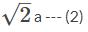From (1) and (2), we can deduce that the square of the diagonal = d2 = 2a2 = 2(area of the square)

Or d =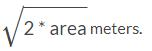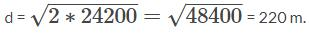QUESTION: 4

A wheel of a car of radius 21 cms is rotating at 600 RPM. What is the speed of the car in km/hr?

Solution:

The radius of the wheel measures 21 cm.
In one rotation, the wheel will cover a distance which is equal to the circumference of the wheel.
∴ in one rotation this wheel will cover 2 * π * 21 = 132 cm.

In a minute, the distance covered by the wheel = circumference of the wheel * rpm
∴ this wheel will cover a distance of 132 * 600 = 79200 cm in a minute.

In an hour, the wheel will cover a distance of 79200 * 60 = 4752000 cm.

Therefore, the speed of the car = 4752000 cm/hr = 47.52 km/hr

QUESTION: 5

A cube of side 5 cm is painted on all its side. If it is sliced into 1 cubic centimer cubes, how many 1 cubic centimeter cubes will have exactly one of their sides painted?

Solution:

When a 5 cc cube is sliced into 1 cc cubes, we will get 5 * 5 * 5 = 125 cubes of 1 cubic centimeter.

In each side of the larger cube, the smaller cubes on the edges will have more than one of their sides painted.
Therefore, the cubes which are not on the edge of the larger cube and that lie on the facing sides of the larger cube will have exactly one side painted.

In each face of the larger cube, there will be 5 * 5 = 25 cubes.
Of these, the cubes on the outer rows will be on the edge. 16 such cubes exist on each face.
If we count out the two outer rows on either side of a face of the cube, we will be left with 3 * 3 = 9 cubes which are not on the edge in each face of the cube.
Therefore, there will be 9 cubes of 1-cc volume per face that will have exactly one of their sides painted.
In total, there will be 9 * 6 = 54 such cubes.

QUESTION: 6

Is triangle ABC with sides a, b and c acute angled?
Triangle with sides a2, b2, c2 has an area of 140 sq cms.
Median AD to side BC is equal to altitude AE to side BC.

Solution:

The statement provides us with one valuable information: we can form a triangle with sides a2, b2, c2.

For any triangle we know that sum of two sides is greater than the third side.

So, we can infer that a2 < b2 + c2.

The inequality above is the condition to be met if the triangle with sides a, b and c were to be an acute triangle.

QUESTION: 7

Is triangle ABC obtuse angled?
a2 + b2 > c2
The center of the circle circumscribing the triangle does not lie inside the triangle.

Solution:

The center of the circle circumscribing the triangle does not lie inside the triangle.

From statement 2 we know that the triangle is not acute. Without knowing whether 'c' is the longest side, we will not be able to conclude whether the triangle is obtuse.

Eliminate choice C.

QUESTION: 8

If 10, 12 and 'x' are sides of an acute angled triangle, how many integer values of 'x' are possible?

Solution:

Finding the answer to this question requires that you know this key property about sides of an acute triangle.

If 'a', 'b', and 'l' are the 3 sides of an acute triangle where 'l' is the longest side then l2 < a2 + b2

The sides are 10, 12, and 'x'.
Scenario 1: Among the 3 sides 10, 12, and x, for values of x ≤ 12, 12 is the longest side.
Scenario 2: For values of x > 12, x is the longest side

Possibilities in scenario 1:

When x ≤ 12, let us find the number of values for x that will satisfy the inequality 122 < 102 + x2
i.e., 144 < 100 + x2
The least integer value of x that satisfies this inequality is 7.
The inequality will hold true for x = 7, 8, 9, 10, 11, and 12. i.e., 6 values.

Possibilities in scenario 2:

When x > 12, x is the longest side.
Let us count the number of values of x that will satisfy the inequality x2 < 102 + 122
i.e., x2 < 244
x = 13, 14, and 15 satisfy the inequality. That is 3 more values.

Hence, the values of x for which 10, 12, and x will form sides of an acute triangle are x = 7, 8, 9, 10, 11, 12, 13, 14, 15. A total of 9 values.

QUESTION: 9

How many diagonals does a 63-sided convex polygon have?

Solution:

The number of diagonals of an n-sided convex polygon =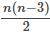This polygon has 63 sides. Hence, n = 63.

Therefore, number of diagonals =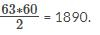QUESTION: 10

What is the radius of the incircle (circle inscribed) of the triangle whose sides measure 5, 12 and 13 units?

Solution:

5, 12 and 13 is a Pythagorean triplet. So, the triangle is a right triangle.

Area using Method 1

Area of a triangle =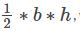where 'b' is the base and 'h' is the height of the triangle.

In this right triangle, if the base is 12, the height will be 5 or vice versa.

In either case, area =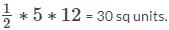QUESTION: 11

If the sum of the interior angles of a regular polygon measures 1440o, how many sides does the polygon have

Solution:

Sum of all interior angles of a convex polygon = (n - 2)* 180

So, (n - 2) * 180 = 1440

n - 2 = 8

n = 10

QUESTION: 12

What is the measure of the radius of the circle that circumscribes a triangle whose sides measure 9, 40 and 41?

Solution:

9, 40 and 41 is Pythagorean triplet. So, the triangle is right angled.
In a right angled triangle, the radius of the circle that circumscribes the triangle is half the hypotenuse.
In the given triangle, the hypotenuse = 41.
Therefore, the radius of the circle that circumscribes the triangle = 41/2 = 20.5 units

QUESTION: 13

What is the area of an obtuse angled triangle whose two sides are 8 and 12 and the angle included between two sides is 150o?

Solution:

If two sides of a triangle and the included angle 'y' is known, then the area of the triangle = 1/2 * (Product of sides) * sin y
Substituting the values in the formula, we get 1/2 * 8 * 12 * sin 150o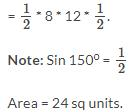QUESTION: 14

Vertices of a quadrilateral ABCD are A(0, 0), B(4, 5), C(9, 9) and D(5, 4). What is the shape of the quadrilateral?

Solution:

The lengths of the four sides, AB, BC, CD and DA are all equal to  √41.
Hence, the given quadrilateral is either a Rhombus or a Square.
The diagonals of a square are equal. The diagonals of a rhombus are unequal.
Compute the lengths of the two diagonals AC and BD.
The length of AC is√162  and the length of BD is √2.
As the diagonals are not equal and the sides are equal, the given quadrilateral is a Rhombus.